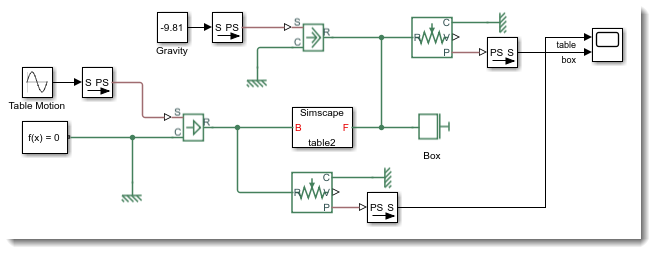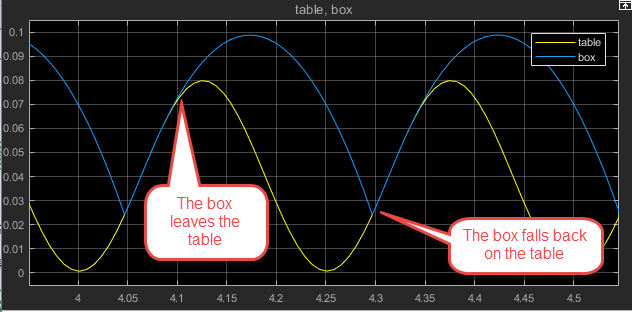# Modecharts: Modeling discrete modes in Simscape

Last week I described how to model a box on a moving table using Simulink subsystems as Stateflow state.

As it is the case for most systems, there are many ways to model this box-table system in Simulink. I thought it would be interesting to model the same system this week using a new Simscape feature: Modecharts

Background

In a way similar to the Simulink Subsystems as Stateflow states, Simscape modecharts are designed to model systems with discrete operating modes.

In our example of a box on a table, the two modes are:

• Locked: The box and the table are moving together at equal velocity, the table is able to generate whatever force is needed to keep their velocity equal.
• Unlocked: The box flies in the air, zero forces are generated between the table and the box

To implement my table-box relationship, I thought it would be a good idea to get inspired by the fundamental friction clutch. If you look at the documentation, you will find the following diagram for the unidirectional case, which is very close to what I need. The only thing I need to change is the condition to lock, which will be function of the position instead of the velocity.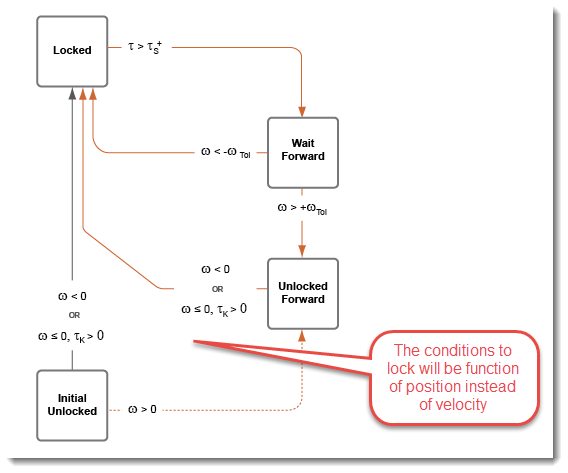Notice that we cannot have only two modes like in the Stateflow case. To avoid the solver chattering between locked and unlocked, we introduce a "waiting" state where we wait after getting out of the locked state to have reached a threshold velocity before reaching the unlocked state.

Mode Charts

Now, how do we implement that in Simscape?

To begin, we use the Simscape language to create a standard component with two ports from the mechanical translational domain. To help with that, I recommend starting with one of the component from the foundation library, for example a spring. This will look like: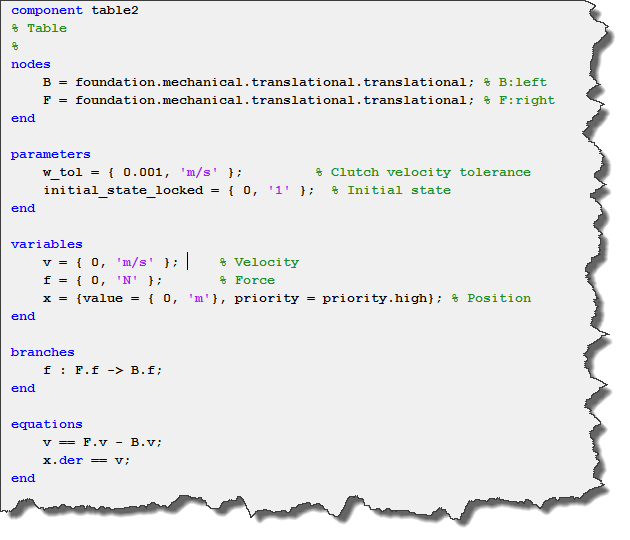Then we can begin defining our modes. In our modecharts, we need to define 3 sections: the modes,
the transitions, and the initial mode.

About the modes, in the case we are locked, the equation we want is the velocity between the two ports to be zero. In all the other modes, the equation we want is the force between the two ports to be zero.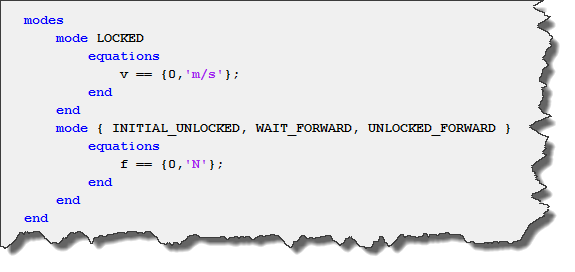For the transitions, for each transition in the above diagram, we specify the source state, the destination state, and the condition when the transition should happen.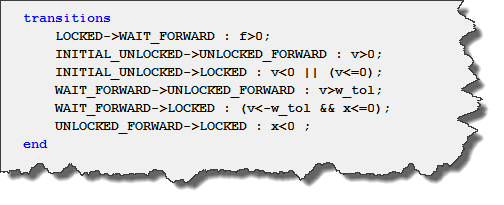Finally, we can specify in which mode the simulation will start. If you do not specify one, the first mode defined will be used.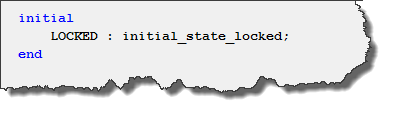Now that our component is complete, we can grab a Simscape Component block and specify the SSC-file we just created.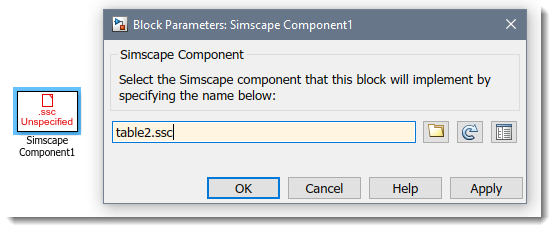To create a simulation similar to last week, we connect an Ideal Velocity Source to the base (this will be our moving base), and a Mass to the other port (this will be our box). Using Motion Sensor blocks, we can confirm that the motion is as expected.